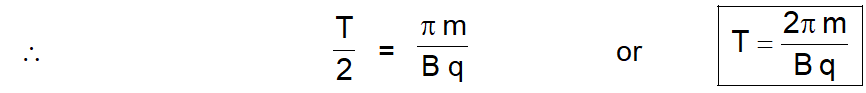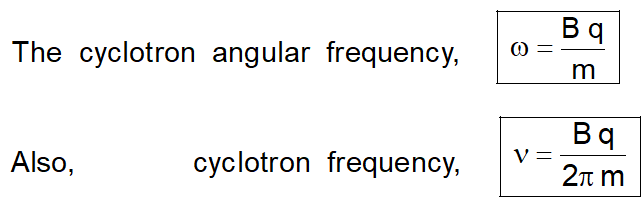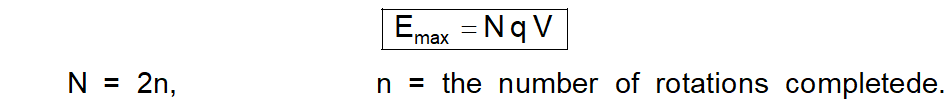Download the eSaral app and start learning from Kota's top IITians and doctors.

# Cyclotron Working Principle, Applications & Frequency || Class 12 Physics Notes

The cyclotron frequency (or, equivalently, gyro-frequency) is the number of cycles a particle completes around its circular circuit every second.

## Cyclotron Frequency

Under the action of the given magnetic field (B fixed), the given positive ion (e/m fixed) will cover the semi-circular path in a fixed time only, if t in equation is equal to $\frac{T}{2}$ Where T is the time period of the electric field.### Maximum energy of the positive ions

Maximum energy acquired by positive ions depends on the radius R of the dees. After acquire this energy positive ion emerge out the dees and use for hitting the target. Let $\mathrm{V}_{\mathrm{max}}$ be the velocity acquired by the positive ions, when it moves along the largest circular path i.e. path of radius equal to the radius R of the dees. Then $\mathrm{B} \mathrm{q} \mathrm{v}_{\max }=\frac{\mathrm{m} \mathrm{v}_{\max }^{2}}{\mathrm{R}}$ OR $v_{\max }=\frac{B q R}{m}$   Therefore maximum kinetic energy gained by the positive ion, $$E_{\max }=\frac{1}{2} m v_{\max }^{2}=\frac{1}{2} m\left(\frac{B q R}{m}\right)^{2}$$ OR $E_{\max }=\frac{1}{2} \frac{B^{2} q^{2} R^{2}}{m}$   The maximum energy acquired by the positive ion can be expressed in another form as given below : If V is the potential difference applied between the dees and N is the number of times, the positive ion cross the gap between the dees before leaving the dees, then $E_{\max }=N q V$ $N=2 n$ $n=$ the number of rotations completede.Also Read:

#### Click here for the Video tutorials of Magnetic Effect of Current Class 12

About eSaral At eSaral we are offering a complete platform for IIT-JEE & NEET preparation. The main mission behind eSaral is to provide education to each and every student in India by eliminating the Geographic and Economic factors, as a nation’s progress and development depends on the availability of quality education to each and every one. With the blend of education & technology, eSaral team made the learning personalized & adaptive for everyone.